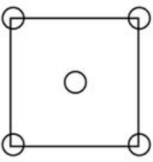# Problem: (a) Derive planar density expressions for  (100) in terms of the atomic radius R. (b) Compute linear density values for the plane for aluminum. (c) Consider the (100) plane in FCC: How many atoms are centered on the  In FCC?

###### FREE Expert Solution

(a)

Planar density, PD = number of atoms centered on the plane/ area

For FCC (100):

The number of atoms = (1/4)(4) + 1 = 2

The area of the plane:

$\begin{array}{rcl}\mathbf{A}& \mathbf{=}& {\mathbf{L}}^{\mathbf{2}}\\ & \mathbf{=}& {\mathbf{\left(}\frac{\mathbf{4}\mathbf{R}}{\sqrt{\mathbf{2}}}\mathbf{\right)}}^{\mathbf{2}}\end{array}$

A = 8R2

97% (251 ratings)###### Problem Details

(a) Derive planar density expressions for  (100) in terms of the atomic radius R.

(b) Compute linear density values for the plane for aluminum.

(c) Consider the (100) plane in FCC:How many atoms are centered on the  In FCC?